# 2nd PUC Physics Question Bank Chapter 10 Wave Optics

Students can Download 2nd PUC Physics Chapter 10 Wave Optics Questions and Answers, Notes Pdf, 2nd PUC Physics Question Bank with Answers helps you to revise the complete Karnataka State Board Syllabus and to clear all their doubts, score well in final exams.

## Karnataka 2nd PUC Physics Question Bank Chapter 10 Wave Optics

### 2nd PUC Physics Wave Optics One Marks Questions and Answers

Question 1.
Name the person who gave the corpuscular model of light and derived Snell’s law of refraction?
Descartes (in the year 1637).

Question 2.
Why is it that corpuscular theory of light is attributed to Isaac Newton?
The corpuscular theory of light was developed further by Isaac Newton in the year 1675. The ‘ theory is attributed to Newton.Question 3.
Who proposed the wave nature of light?
Christian Huygens put forward longitudinal mechanical wave model of light in the year 1678.

Question 4.
Who experimentally verified that the speed of light in water is less than the speed of light in air?
Foucault (in 1850).

Question 5.
Name the physicist who established the wave nature of light.
Thomas Young (1801) discovered the phenomenon of interference of light waves.

Question 6.
Inspite of the wave nature of light, why is that light assumed to travel in a straight line?
The wavelength of light lies in the range of 4000 A to 8000 A. The size of the lens or mirror is much larger than the wavelength. Therefore light may be assumed to travel in a straight line.

Question 7.
Name the branch of optics which neglects the wavelength (λ → 0 )of light.
Geometric optics neglects the finiteness of wavelength of light (but only considers the ray as the path of luminous flow of energy).

Question 8.
Who put forward electromagnetic wave theory of light?
James Clerk Maxwell.

Question 9.
What is a wavefront? (March 2014)
A wavefront is defined as a surface of constant phase (which also represents the locus of points at which particle of the medium have the same state of vibration and are at the same distance from the source of the disturbance).

Question 10.
What is the shape of a wavefront at a large distance away from a point source?
Plane wave front.Question 11.
What is the direction of rays with respect to plane wave fronts?
The rays are at right angles to the plane wavefronts.

Question 12.
What is the shape of a wavefront due to a spherical or point source?
Spherical.

Question 13.
What is a primary disturbance?
A primary source of light is referred to as a primary disturbance.

Question 14.
What are wavelets?
Waves sent out from secondary sources of disturbances are called wavelets.

Question 15.
What constitutes secondary wavefront?
An envelope of wavelets given out by secondary sources having the same phase and distance from the primary wavefront constitutes a secondary wavefront.

Question 16.
What is interference of light? (March 2015)
Interference of light is that phenomenon in which there is a redistribution of energy at a point of superposition of two or more number of waves traveling in the same direction.

Question 17.
Who demonstrated Interference of light for the first time?
Thomas young in the year 1801 demonstrated the Interference of light.

Question 18.
State the condition for destructive interference in terms of path difference between
the two waves. (March 2015)
The path difference between the two waves should be (2n + 1) $$\frac{\lambda}{2}$$ where ‘iT is an integer.

Question 19.
State the condition for constructive interference in terms of path difference between the two waves. (March 2015)
The path difference between the two waves should be nλ where ‘n’ is an integer.

Question 20.
State the condition for constructive interference in terms of phase difference between the two waves.
The phase difference between the two waves should be 2nπ where ‘n’ is an integer.Question 21.
State the condition for destructive interference in terms of phase difference between the two waves.
The condition for destructive interference between the two waves should be (2n + 1) where V is an integer.

Question 22.
What is fringe width?
The distance between two consecutive bright fringes or two consecutive dark fringes is known as fringe width.

Question 23.
How does fringe width vary with wave length?
The Fringe width is directly proportional to the wavelength (β ∝ λ).

Question 24.
How does fringe width vary with slit separation?
Fringe width is inversely proportional to slit separation.

Question 25.
How does fringe width vary with distance between the double slit and the screen?
Fringe width is directly proportional to distance between the double slit and the screen.

Question 26.
What are coherent sources of light?
Two sources of light having either a constant phase or phase difference between them are called coherent sources.

Question 27.
What is the principle behind young’s double slit experiment in obtaining coherent sources?
The principle used is division of amplitude.

Question 28.
Does the law of conservation of energy hold good at the point of destructive interference?
Yes, it does.

Question 29.
Calculate phase difference between the two waves corresponding to a path difference of (λ/6).
Phase difference will be $$\frac{1}{6}$$ (2π) = $$\frac{\pi}{3}$$.

Question 30.
Draw intensity pattern of light in an interference of light waves. Intensity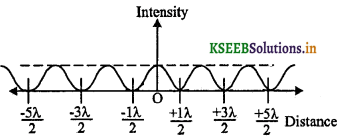Question 31.
What is diffraction of light?
The phenomenon of bending of light around the sharp edge of an obstacle and its encroachment into the region of geometrical shadow is known as diffraction of light.

Question 32.
Who discovered the phenomenon of diffraction of light?
Grimaldi.

Question 33.
State the condition for diffraction of light to take place.
Diffraction of light takes place if the size of the obstacle is of the order of the wavelength of light.

Question 34.
Give the condition for diffraction minima.
The condition for diffraction minima is nλ = d sin θ where, n = 1,2, 3,
θ – is the semi angular width, d – width of the single slit, λ – wavelength of light used.

Question 35.
Give the condition for diffraction secondary maxima.
The condition for diffraction secondary maxima is (2n + 1) $$\frac{\lambda}{2}$$ = d sin θ.
Where, n – 1, 2, 3,

Question 36.
Express angular width of central maximum in terms of wavelength.
For a diffraction minima nλ = d sin θ, to find the width of the central maximum, put n = 1 then sin θ = $$\frac{\lambda}{d}$$: θ = sin-1 = $$\left(\frac{\lambda}{d}\right)$$ Here ‘θ’ is semi angular width.
For a total angular width θ’ = 2θ = 2 sin-1 = $$\left(\frac{\lambda}{d}\right)$$

Question 37.
Give the expression for linear width of central maximum.
Linear width of central maximum = $$2\left(\frac{\lambda D}{d}\right)$$

Question 38.
How does the width of central maximum vary with the wavelength of light used?
The width of central maximum is directly proportional the wavelength of light used.

Question 39.
How does width of central maximum vary with the width of wavelength of light used?
Central maximum is inversely proportional to the width of the slit.

Question 40.
Compare the first secondary maximum with the intensity of central maximum.
IFSM : ICM :: 1 : 22Question 41.
Compare the second secondary maximum with the intensity of central maximum.
IFSM : ICM :: 1 : 61

Question 42.
What type of wave front is used in Fraunhoffer diffraction? ‘
Plane wave front.

Question 43.
What is the resolving power of an instrument?
The reciprocal of the minimum linear separation or angular separation between two point objects at which their diffraction images are just resolved is known, as the resolving power.

Question 44.
For which coloured light, is the angle of diffraction the least?
Violet colour.

Question 45.
Define limit of resolution of a microscope.
The minimum linear separation between two point objects at which they are just resolved is known as limit of resolution of a microscope.

Question 46.
Define limit of resolution of a telescope.
Limit of resolution of a telescope may be defined as the minimum angular separation between the two point objects at which their images are just resolved.

Question 47.
How does resolving power of microscope depend on the wavelength?
RP ∝ $$\frac{1}{\lambda}$$ where, ‘λ’ is the wavelength of light.

Question 48.
How does the resolving power of a microscope depend on the refractive index of the medium between the object and objective?
R.P ∝ n ; where ‘n’ is the R.I. of the medium

Question 49.
How does the resolving power of a telescope depend on the diameter of the aperture?
R P ∝ D; where, ‘D’ is the diameter of the aperture.

Question 50.
What is meant by polarisation?
The phenomenon of confining the vibrations of the electric vector in a specific direction perpendicular to the direction of wave motion is called polarisation.Question 51.
Can longitudinal waves be polarised?
No. Longitudinal waves cannot be polarised.

Question 52.
Name the phenomenon of light that supports the transverse wave of nature.
The phenomenon of light that supports the transverse wave nature of light is polarisation.

Question 53.
Draw a plane polarised light.Question 54.
What is plane of vibration?
A plane of vibration is that plane which contains the vibration of electric vector at right angles to the direction of wave motion.

Question 55.
What is plane of polarisation?
A plane at right angles to the plane of vibration is called as the plane of polarisation.

Question 56.
What is an unpolarised light?
The superposition of waves produced by individual atomic or molecular sources and the electric field vector symmetric about the direction of propagation of wave is known as the unpolarised light.

Question 57.
How can we represent an unpolarised light?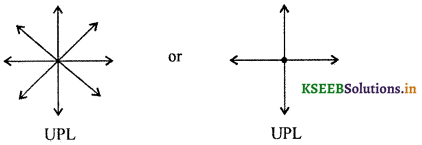Question 58.
How can we represent a plane polarised light?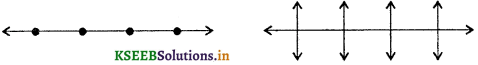Question 59.
Mention any one method to produce plane polarised light.
Reflection.
The other methods are by refraction, double refraction, scattering and selective absorption.

Question 60.
State Brewster’s law.
The tangent of the polarising angle of incidence is equal to the refractive index of the medium.
n = tan θpQuestion 61.
What is polarising angle?
Polarising angle is that angle of incidence for which the reflected ray is completely plane polarised.

Question 62.
What is the angle between the reflected and refracted rays at polarising incidence?
The angle between the reflected and refracted rays will be equal to 90° at the polarising incidence.

Question 63.
Define principal section or principal plane of a crystal.
A plane containing the optic axis and perpendicular to the opposite faces of the crystal is called principal section or principal plane of the crystal for that pair of faces.

Question 64.
What is a polarizer?
A polarizer is an optical medium that plane polarises an unpolarised light passing through it.

Question 65.
What is a detector?
An analyser (detector) is an optical medium that detects the plane polarised light.

Question 66.
Define plane polarised light.
The direction of vibration of electric field vector lies in the plane of sheet of paper. This is called as plane polarised light. The direction vibration of light and its magnitude remains the same.
eg: (a) The blue colour of the sky is a scattered light which is a plane polarised light.
(b) In a double refraction, OL and EOL both are PPL at right angles to each other.

Question 67.
In propagation of electromagnetic waves, what is the angle between the direction of propagation of the wave and the plane of polarisation?
Direction of the propagation of wave and the plane of polarisation are at right angles to each other.

Question 68.
What is a polaroid?
Polaroid is a device made out of synthetic crystalline material in which tiny crystals are aligned parallel to each other and exhibit the property of dichroism.

Question 69.
State Malus law. [March 2014]
If at any instant the axis of a polarizer subtends an angle ‘θ’ with the direction of vibration of incident light, then the intensity of the emergent light I = I0cos2θ, where I0 is the maximum intensity corresponding to θ = 0°.

Question 70.
Say whether radio signals are plane polarised or circularly polarised?
Radio signals transmitted to a satellite are circularly polarised radio waves in which electric fields are rotated.Question 71.
What properties are attributed to ether medium?
Ether medium is suppose to possess very high elasticity and low density.

Question 72.
What is ether?
Ether is a hypothetical medium assumed by Huygens to explain the nature of light.

Question 73.
Mention any one demerit of Huygens’ wave theory of light.
Huygens assumed the presence of the hypothetical medium called ether in his wave theory of light. However the existence of ether medium was later disproved by Michelson and Morley in the year 1887.

Question 74.
Mention any one demerit of Newton’s corpuscular theory of light.
Newton’s corpuscular theory suggested that velocity of light in a denser medium is greater than in a rarer medium. Foucault and Michelson experimentally verified that light in denser medium has lower velocity than in a rarer medium.

Question 75.
How did Newton account for different colours of light?
Newton assumed that different colours are due to different sizes of corpuscles.### 2nd PUC Physics Wave Optics Two Marks Questions and Answers

Question 1.
When are two identical waves said to interfere (a) constructively (b) destructively?
(a) Two identical waves when interfere constructively, result in maximum intensity
(Imax = 4a2 where‘a’is the amplitude)

(b) Two identical waves when interfere destructively, result in a zero or minimum intensity
(Imin = 0).

Question 2.
Give any two characteristics of interference of light waves.
Characteristics of interference of light waves are,

1. Bright fringes are equally bright and dark fringes are equally dark.
2. Fringe width remains uniform for either bright or dark fringes.

Question 3.
Compare width of slits with the intensity and hence amplitude of the waves.
Width α a2 and Intensity α a2.Question 4.
If the apparatus used in YDSE is immersed in water then what will happen to the fringe width.
Fringe width decreases because β’ = $$\frac{\beta}{n}$$ where V is the medium .

Question 5.
A thin glass plate is introduced in front of one of the double slits. What will happen to the fringe width!
Fringe width will remain the same and will be shifted by (µ – 1) t where ‘µ’ is R.I. of the medium and ‘t’ is the thickness of the medium.Question 6.
Draw a neat labelled diagram of diffraction image of an object.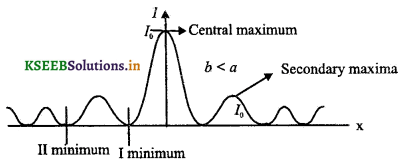Question 7.
Draw a neat diagram of experimental set up for Fraunhoffer diffraction due to a single slit.Question 8.
Draw parallel and crossed polaroids neatly.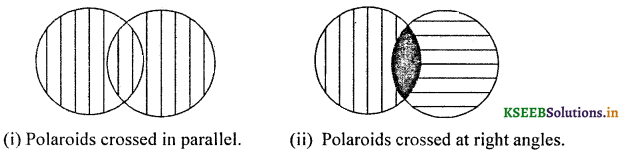Question 9.
Name the scientists associated with the discovery of
1. Polarisation by reflection
2. Birefringence.
1. Etienne Louis Malus in the year 1809.
2. Erasmus Bartholinus in the year 1669.

Question 10.
What is meant by an unipolarised wave?
If the displacement of the point of a wave changes randomly with time at right angles to the direction of propagation then such wave is called unipolarised wave.Question 11.
Draw a neat labelled diagram to show the real image formed by the objective lens of the microscope.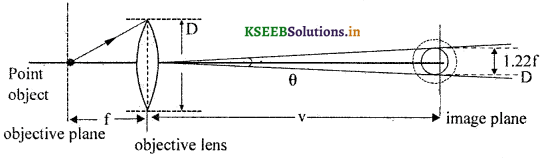Question 12.
What is meant by red shift and blue shift?
If the source of light moves away from the observer on Earth, then the wavelength of light observed shifts towards the higher wavelength. This shift of wavelength is called red shift. If the source of light moves towards the observer on Earth, then the wavelength of light shifts towards the lower wavelength. This shift is known as the blue shift.

Question 13.
In what way is diffraction from each slit related to interference pattern in a double slit experiment?
The intensity of interference fringes in a double slit arrangement is modulated by the diffraction pattern of each slit.

Question 14.
When a tiny circular obstacle is placed in the path of light from a distant source, a bright spot is seen at the centre of the shadow of the obstacle. Explain why?
Waves diffracted from the edge of the circular obstacle interfere constructively at the centre of the shadow producing a bright spot.
If crest of one wave falls on the trough of the other wave, then it results in destructive interference.

Question 15.
Give the expression for limit of resolution of a microscope along with the meaning of the symbols used. (July 2014)
Limit of resolution of a microscope is given by dx = $$\frac{\lambda}{2 n \sin \theta}$$

where, ‘λ’ is wavelength of light used to illuminate the point objects, ‘n’ is the refractive index of the behind between the object and the objective and ‘θ’ is the semi vertical angle subtended at the object by the objective. Resolving power can be increased by placing a retracting medium between the object and the objective and by illuminating the object by lower wavelength of light like ultraviolet light.

Question 16.
Give the expression for limit of resolution of a telescope along with the explanation of the symbols used.
dθ = $$\frac{1.22 \lambda}{D}$$
where, ‘λ’ wavelength of light emitted by the distance source/object
D – diameter of the aperture.

### 2nd PUC Physics Wave Optics Three Marks Questions and Answers

Question 1.
State any three conditions for a sustained interference of light waves.
For a sustained interference of two light waves,

1. Both the sources should be identical (same amplitude and frequency).
2. Both the sources should be coherent (same phase or phase difference between the two waves).
3. Both the waves should travel in the same direction and interfere at small angles.
4. Both the sources should be separated by only a small distance.
5. Both the sources should be of narrow thickness.

Question 2.
Give the formula for the linear separation of bright and dark fringes.
(a) For bright fringes x = ± n β; n = 1,2, 3,4,
(b) For dark fringes x = ±(2n + 1)$$\frac{\beta}{2}$$ Where β = $$\frac{\lambda D}{d}$$ and n = 0, 1, 2, 3,…..Question 3.
Give the formula for angular separation of bright and dark fringes.
(a) For bright fringes, semi angular width is given by θ = sin-1 $$\left(\frac{n \lambda}{d}\right)$$ where n = 1, 2,3, …
(b) For dark fringes, semiangular width is given by θ = sin-1 $$\left(\frac{(2 n+1) \lambda D}{2 d}\right)$$
where, n = 0, 1, 2, 3, …….

Question 4.
Compare Imax and Imin with amplitudes of the interfering waves.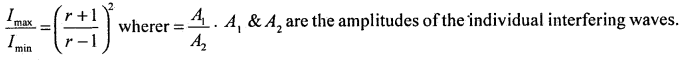Question 5.
Explain Doppler Effect of light based on wavefronts?
The time taken between the arrival of two wavefronts will be longer at the observer than it is at the source. Hence when the source of light moves away from the observer, the wavelength of light from the source increases. This is known as the Doppler Effect (red shift)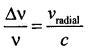where, ‘c’ – velocity of light in vacuum
vr – radial speed of source of light
Vradiai is positive when the s&urce is moving away from the observer and is -ve when the source is moving towards the observer.

Question 6.
Write any three practical applications of polaroids.

1. Polaroids are used to produce and detect plane polarised light.
2. Polaroids are used in photographic cameras to eliminate unwanted portion of light.
3. Polaroids ar&used as microscope objectives to eliminate glare of light from the minute particles under observation.

Other applications:

1. Polaroids are used in the construction of Laurentz half shade polarimeter.
2. Polaroids are used to improve the colour contrast in the old paintings.Question 7.
Explain the refraction of plane wavefront of light through a prism.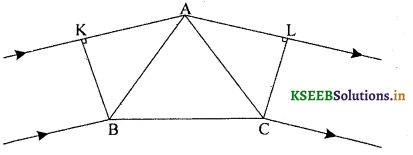The secondary wavelets from B will travel almost the whole distance inside the prism to reach the point C. But the secondary wavelets from K will travel the whole distance in air before it is incident on the prism at A. The points K and B are in phase, and so do L and C. LC and KB represent the plane wavefronts.

Question 8.
Explain the refraction of plane wavefront of light through a convex lens.Let AB represent a plane wavefront. The secondary wavelets from A and B reach the points A’ and B’ respectively in. same the time as the secondary wavelets reach from P1 and P2. Thus the points A’, P2 and B’ are in the same phase. This explains why a parallel beam of lights on refraction through a convex lens gets converged and gives a spherical wavefront, whereas the plane wavefront diverges in the case of a concave lens.Question 9.
Explain the refraction of plane wavefront of light in a concave mirror.In the figure, AOB represents a plane wavefront. A’O’B’ represents a converging spherical wavefront and XPX’ represents a concave mirror. The time of travel of a beam AX and BX’ will be the same and shorter than for the light beam OP. Thus the emergent wavefront is a converging spherical wavefront. Reflection of a plane wavefront from a concave mirror

### 2nd PUC Physics Wave Optics Five Marks Questions and Answers

Question 1.
Prove Snell’s law of refraction by using Iluygens’ concept of plane wavefronts.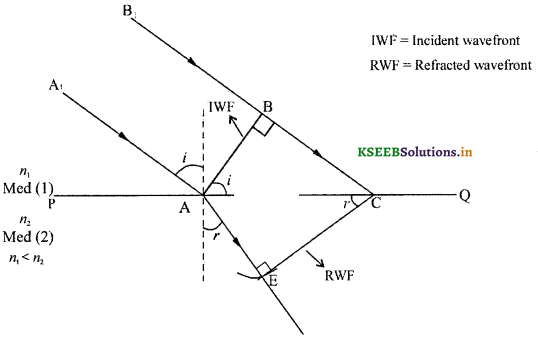Distance AE = v2τ ; τ – time taken for optical path
Distance BC = v1τ; i1 – angle of incidence in medium (1)
From the right angled ∆ ABC, sin i = $$\frac{B C}{A C}$$
and from the right angled ∆ AEC, sin r = $$\frac{\mathrm{AE}}{\mathrm{AC}}$$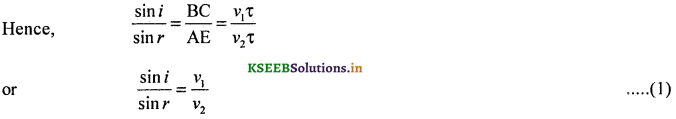By definition, absolute R.I of a medium w.r. to air/vacuum = n = 
for medium (1) = n1 = $$\frac{c}{v_{1}}$$
for medium (2) = n2 = $$\frac{c}{v_{2}}$$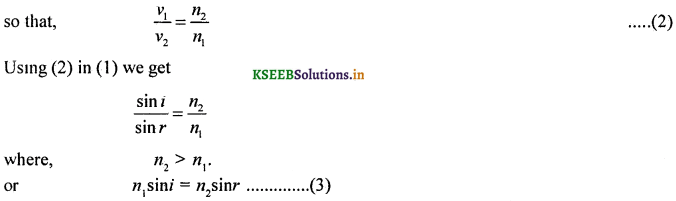i.e., R.I. of medium (1) times sine of angle in the medium (1) = R.I of medium (2) times sine of angle in the medium 2.
This expression (3) is known as the Snell’s law.
The ratio $$\frac{n_{2}}{n_{1}}$$ can be written as n2 (R.I. of medium (2) w.r.t medium (1)).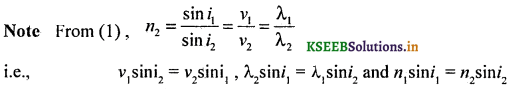Question 2.
Explain refraction of light from a denser to a rarer medium, using the concept of wavefronts.where, BC = v1τ, AD = v2τ, v2 > v1, τ = time taken to travel optical path by light.
AB = incident wave front
DC = refracted wave front.
i = angle of incidence in medium (1)
For, i ≥ ic, total internal reflection takes place at the interface PQ.
For z < ic, there will be refraction of light from denser to rarer mediumQuestion 3.
Explain reflection of a plane wave by a plane surface.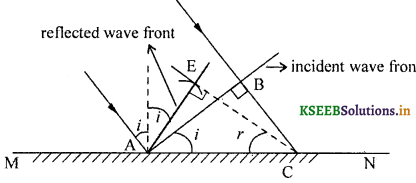Let ‘V’ be the speed of wave in the medium, t be the time taken by the wavefront to move
from the point B to C.
BC = vτ
Let AE be the radius of an arc drawn at E and EC be the tangent drawn at E.
AE = BC = vτ
The triangles AEC and CBA are congruent and therefore î = r̂ Hence the law of reflection is proved.

Question 4.
Describe an experimental set up to Young’s double slit experiment.
A fine slit is etched on a cardboard through which monochromatic light (such as from a sodium vapour lamp) is allowed to pass through. The width.of the slit is about 1 mm.

Another card board is placed at a distance of 10 cm. (a) from the first. Two fine slits separated within a distance of 2 mm and about 0.5 mm in thickness each are etched out. The second cardboard serves as a double slit.

A screen is placed at a distance of 1 m (D) from the double slit. When the light is switched on, an alternate bright and dark bands of lights are observed on the screen. All the bright bands are of uniform thickness are equally spaced and are of uniform brightness.

All dark bands are equally dark and are spaced at equal distances. These alternate bright and dark bands of light are called fringes.

The consecutive distance between any two dark fringes or bright fringes is called fringe width and the fringe widths of dark or bright fringes are equal.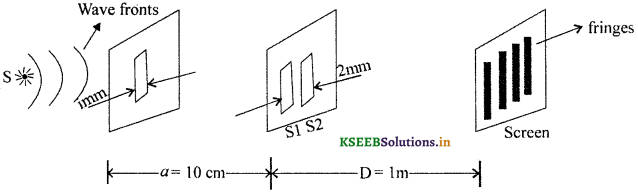Conditions: When crest (trough) of one wave from ‘S1‘ and crest (trough) of another wave front ‘S2‘ fall on each other then constructive interference takes place.Question 5.
Obtain an expression for the fringe width in an interference obtained in the double slit experiment. (March 2014, July 2015)
Let A and B be two slits separated by a distance ‘d’. Let ‘λ’ be the wavelength of light. Let ‘D’ be the distance between the screen and the double slit.
Let ‘C’ be a point on BP such that AP ≈ CP.
Path difference between the two waves reaching ‘P’ is given by BP – AP = BC = δ.
From the Δ BFP, BP2 = BF2 + FP2 and from the δ ΔAEP, AP2 = AE2 + EP2
BP2 – AP2 = (D2 + FP2) – (D2 – EP2)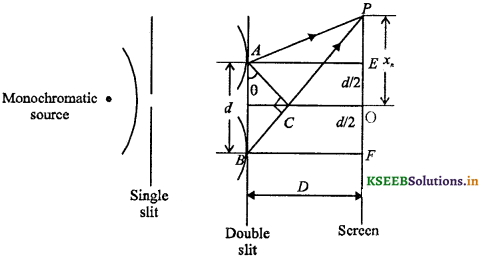For a point ‘P’ close to ‘O’,
BP ≈ AP= D
(BP – AP) (2D) = 2x.d.
But (BP – AP)BC = δ
i.e., δ = $$\frac{x d}{D}$$
or x = $$\frac{\delta D}{d}$$
For a constructive interference δ = nλ.
Distance of nth bright fringe from the central bright fringe xn = $$n \frac{\lambda D}{d}$$
Distance of (n + 1)th bright fringe from the central bright fringe xn + 1 = (n + 1)$$\frac{\lambda D}{d}$$
By definition, Fringe width is the distance between two consecutive bright or dark fringes.Fringe width β ∝ D, β ∝ λ and β ∝ $$\frac{1}{d}$$
We can show that the fringe width between any two dark fringes is also β = $$\frac{\lambda D}{d}$$.Question 6.
Show that two waves interfere constructively when the path difference between them is an integral multiple of wavelength.
Let, Y1 = A1 sin (ωt – Φ1) and Y2 = A2 sin (ωt – Φ2) when these waves interfere, the resultant displacement will be Y = Y1 + Y2
i.e., Y = A1 sin (ωt – Φ1) + A2 sin (ωt – Φ2).
i.e., Y = A sin (ωt – Φ)For a constructive interference, A = Amax.
The implies that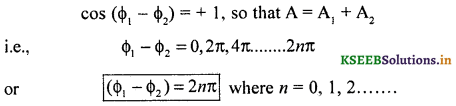For a constructive interference, path difference δ = nλ

Question 7.
Show that two waves interfere destructively when the path difference between them is an odd multiple of half wavelength.
Let Y = A sin (ωt – Φ1) and Y2 = A sin (ωt – Φ2) represent two interfering waves.
Their resultant displacementQuestion 8.
S.T. for a constructive interference of two identical and coherent light waves, the maximum intensity is four times the intensity of individual waves and zero for a destructive interference.
For an interference of two identical waves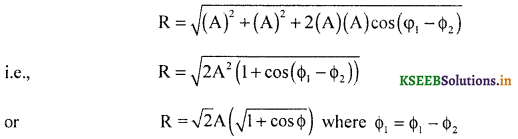Question 9.
Give an account of the analysis of Fraunhoffer diffraction due to a single slit.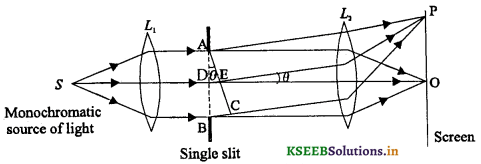Let the path difference between the secondary waves between A and C be ‘λ’ Let ‘D’ be the mid point of the wave front. The path difference between the points ‘DE’ and ‘BC’ will be $$\frac{\lambda}{2}$$. In effect destructive interference takes place at ‘P’.
Therefore for nλ = d sin θ where n = 1,2, 3 ……. divisions of wave front will be $$2\left(\frac{\lambda}{2}\right), 4\left(\frac{\lambda}{2}\right)$$ ……. for n = 1, 2,…… However if the division of wave fronts is $$3\left(\frac{\lambda}{2}\right), 5\left(\frac{\lambda}{2}\right)$$ ……. for n = 1, 2, 3, ….. then (2n + 1) = d sin 0 determines the condition for constructive interference and d is width of the single slit.Question 10.
Prove Brewster’s Law.As an unpolarised light enters the denser medium, a part of light at the point of incidence gets reflected and the rest refracted in the dense medium. It is noticed that the refracted beam of light is either partially polarised or completely Rarer unpolarized. However, the reflected beam gets completely polarised in the plane at right angles E to the plane of incidence at a particular angle of Denser incidence called polarising angle.

It is also noticed that the angle between the completely polarised reflected beam and the refracted beam are at right angles to each other. Applying Snell’s law of refraction, we write absolute refractive index, (rarer medium is taken as air)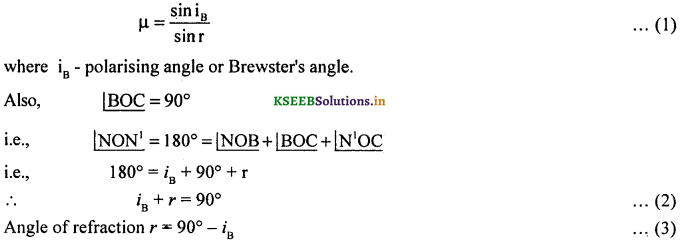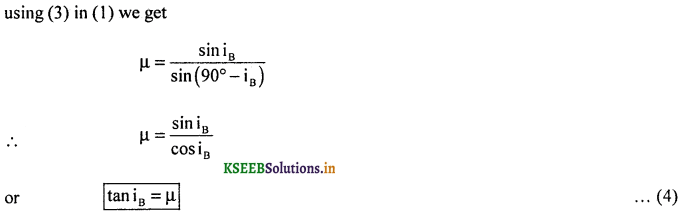Expression (4) is known as the Brewster’s law. The tangent of polarising angle for air-medium interface is equal to the absolute refractive index of the medium for other than air-medium interface.Note : David Brewster, in the year 1811, discovered that at the polarising angle of incidence, the reflected light is completely plane polarised.

Question 11.
Distinguish between interference and diffraction of light.### 2nd PUC Physics Wave Optics List of Formulae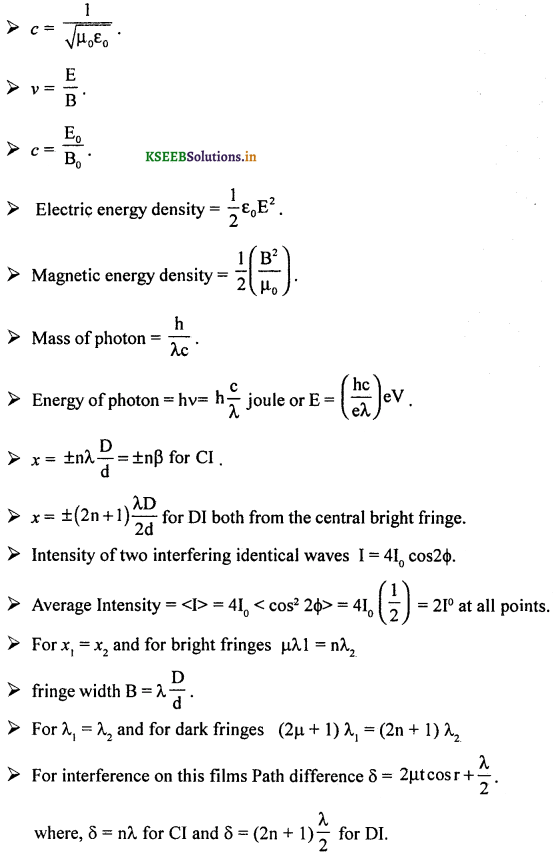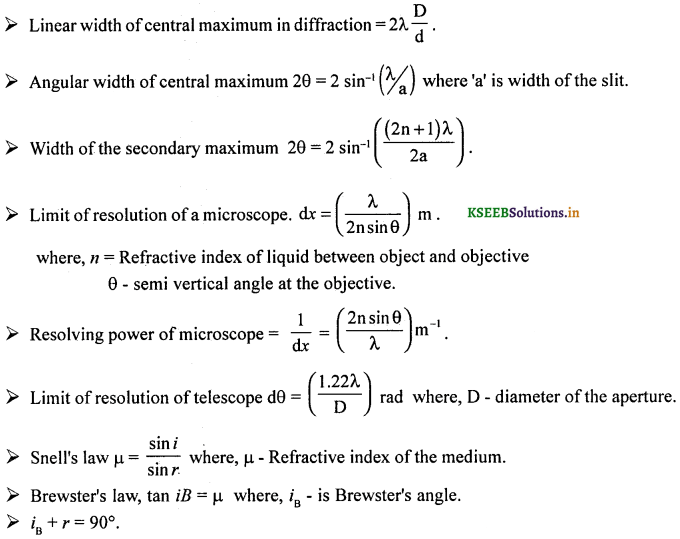### 2nd PUC Physics Wave Optics Numericals and Solutions

Question 1.
In young’s double slit experiment using a source of light of wavelength 5000 Å, the bandwidth obtained is 0.6 cm. If the distance between the screen and the slit is reduced to half, what should be the wavelength of the source to get fringes 0.003m wide?
Given λ = 5000 Å = 5 × 10-7                                                                                                                                                                             m
β1 = 0.6 cm = 6 × 10-3 m
β2 = 0.003 m = 3 × 10-3 m, D2 = $$\frac{D_{1}}{2}$$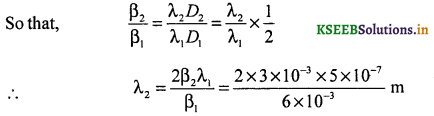i.e., λ2 = 5 × 10-7 m = 5000Å
Wave length of light should be 5000 Å.Question 2.
In young’s double slit experiment, two slits 0.18 mm apart are illuminated by a light of wavelength 589.3 nm. Calculate the distance of (i) 5th bright and (ii) 3rd dark fringes from the midpoint of the interference pattern obtained on a screen kept 0.6 m away from the slits.
Given that d = 0.18 × 10-3 m
λ = 589.3 × 10-9 m, D = 0.6 m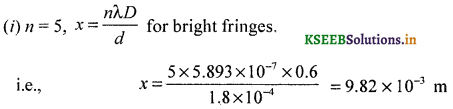(ii) n = 2 for 3rd dark fringe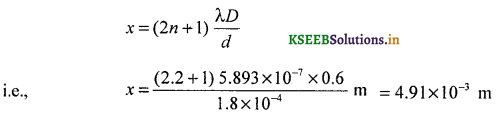Question 3.
In young’s double slit experiment, the total width of 10 fringes is observed as 2 × 10-3m and the slits are separated by a distance of 2 × 103m. Find the distance between the slits and the screen, when a light of wavelength 6000A is used.
Given 10 β = 2 × 10-3 m so that β = 0.2 × 10-3 m
d = 2 × 10-3, λ = 6 × 10-7 m, D = ?Question 4.
In young’s double slit experiment, distance between the slits ¡s 1mm. The fringe width is found to be 0.6 mm. When the screen is moved throügh a distance of 0.25 m, the fringe width becomes 0.75 mm. Find the wavelength of light used. (March 2015)
Given d = 1 × 10-3 m, β = 6 × 10-4m, D’ = D + 0.25
β’ = 7.5 × 10-4m λ = ?i.e., 5D = 4D + 1
∴ D = 1m
Substitvting the values of β, d and D,i.e., λ = 6 × 10-7 m
or λ = 6000 Å

Question 5.
Calculate the distance between the centers of 4th and 7th bright fringes in an interference pattern produced in young’s slit experiment. Given separation between the slits = 1.1 × 10-3, wavelength of light used = 589.3 nm, and distance of the screen from the double slit = 1.3m.
Given d = 1.1 × 10-3 m, λ = 589.3 × 10-9m, D = 1.3 m
We know that distance of the nth bright fringe from the central fringe = x = $$\frac{n \lambda D}{d}$$
Hence distance between 7th and 4th bright fringes will be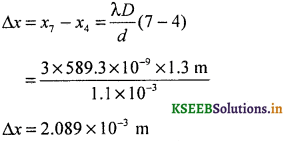Question 6.
Light of wavelength 6000 Å is used to obtain interference fringe of width 6 mm in a young’s double slit experiment. Calculate the wavelength of light required to obtain fringe of width 4 mm if the distance between the screen and slits is reduced to half of its initial value.
Given λ = 6 × 10-7 m, β = 6 × 10-3 m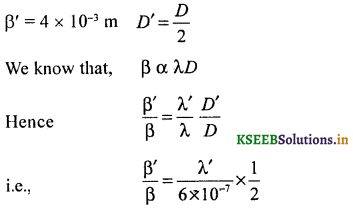Question 7.
Calculate the fringe width of the interference pattern. Given wavelength of light used = 678 nm, distance between the slits = 0.35 mm, distance between the screen and the double slit = 1 m.
Given λ = 678 × 10-9 m, d = 3.5 × 10-4 m
D = 1 m
We know that the fringe width β = $$\frac{\lambda D}{d}$$Fringe width obtained will be 1.937 × 10-3 m.

Question 8.
A beam of light consisting of two wavelengths 500 nm and 400 nm is used to obtain interference fringes in Young’s double slit experiment. The distance between the slits is 0.3 mm and the distance between the slits and the screen is 1.5 m. Compute the least distance of the point from the central maximum, where the bright fringes due to both the wavelengths coincide.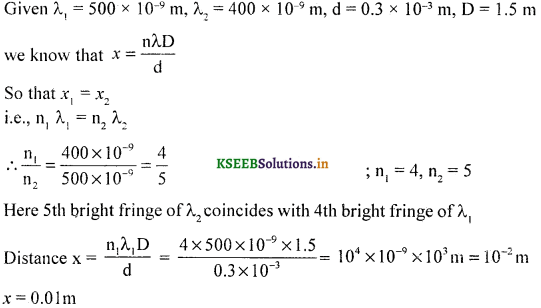Question 9.
Two towers on top of the two hills are 40 km part. The line joining them passes 50 m above the hill half way between the towers. What is the longest wavelength of radio waves, which can be sent between the towers without appreciable diffraction effects?
Maximum wave length,
λ = $$\frac{D}{a}=\frac{50}{40 \times 10^{3}}$$
= 1.25 × 10-3 m = 12.5 cm

Question 10.
A parallel beam of light of wavelength 500nm falls on a narrow slit and the resulting diffraction pattern is observed on a screen 1m away. It is observed that the first minimum is at a distance of 2.5 nm from the centre of the screen. Find the width of the slit.
Given I = 5 × 10-7 m, D = 1m, x = 2.5 × 10-3Question 11.
The diameter of the objective of a telescope is 1.2m. If the wavelength of light used is 546 nm, then calculate the limit of resolution of the telescope.
Given D = 1.2m, λ = 546 × 10-9 m
Limit of resolution,Question 12.
Calculate the resolving power of a telescope whose limit of resolution is 2.44 × 10-6 rad.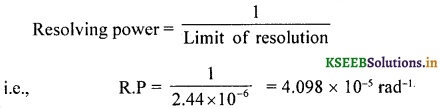Question 13.
In an experiment with a microscope, light of wave length 4240 Å is used. The limit of resolution is found to be 3 × 10-7. What is the semi vertical angle?
Given λ = 4.24 × 10-7 m, dx = 3 × 10-7m
Resolving power = Limit of resolutionQuestion 14.
Calculate the resolving power of a microscope, whose limit of resolution is 2.4 × 10-4m.
Resolving power of a microscope = $$\frac{1}{d x}$$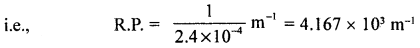Question 15.
The semi vertical angle subtended by two points of an object at the objective of a microscope is 30°. The object is illuminated using light of wavelength 589.3 nm. Calculate the minimum distance between the two points if these are just resolved. If a medium of R.I 1.48 is used between the object and the objective; then calculate the new limit of resolution. Comment on the improvement in the limit of resolution.
Given θ = 30°, λ = 5.89 × 10-7 m.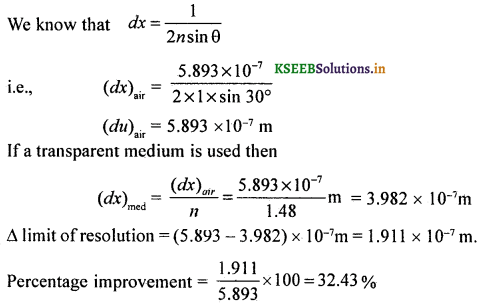i.e., the instrument can resolve even if the distance between the points is decreased by 32.43%.

Question 16.
If the limit of resolution of the eye is 1′ of an arc then calculate its resolving power.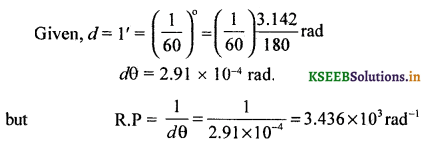Question 17.
A telescope of aperture 0.02 m is used to focus the two head lamps 1.2m apart. If the wavelength of light emitted by the automobile is 589.6 nm then calculate the resolving power of the telescope. If the R.P of the eye is 3.436 × 103 rad-1, then calculate the magnifying power of the telescope
Given D = 0.02 m, x = 1.2m, λ = 589.6 nm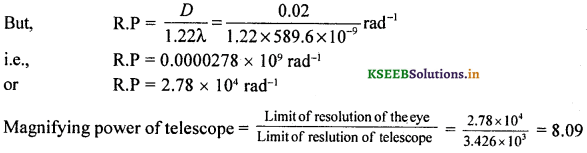Magnifying power of the telescope is eight times that of the eye.

Question 18.
A telescope of aperture 0.15 in is used to view two heavenly bodies separated by a certain linear distance. If the distance of these two is 1 million light years from the earth and the wavelength of light emitted is 440 nm, then calculate the linear separation between them.
Solution:D = 0.15 m, λ = 440 × 10-9mdθ = 35.79 × 10-7 rad
since x = ydθ
i.e., x = 106 × 35.79 × 10-7
i.e., x = 3.579 light years.

Question 19.
The angular width of central maximum is 0.1° If the width of the single slit is 10-5 m, then calculate the wavelength of light used.
2θ = 0.1°
∴ θ = 0.05°, d = 10-5m, λ = ?, n = 1
Condition for minimum nλ = d sin θ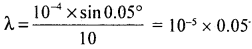or λ = 5 × 10-7 m = 5000 Å

Question 20.
Calculate the angular width of the 2nd secondary maximum and fourth dark fringe in the diffraction pattern obtained due to a single slit of width 10-5m illuminated by a wavelength of light 541 nm.
(i) For Cl, (2n + 1)$$\frac{\lambda}{2}$$ = d sin θsin θ = 0.1352, for small angle,
θ = 0.1352 rad (≈ 8°)
angular width = 2θ = 0.2704 rad.

(ii) For DI, nλ = d sin θ
4 × 541 × 10-9 % = 10-5 sin θ
sin θ = $$\frac{2164 \times 10^{-9}}{10^{-5}}$$
sin θ = 2164 × 10-4 = 0.2164
or θ ≈ 12°Question 21.
Refractive index of glass is 1.5. Find the polarising angle for air-glass interface.
tan iB = µ
iB = tan-1 (1.5000) = 56°18′

Question 22.
Polarising angle for water is 53 8′. Find the critical angle for air-water interface.
tan iB = µ
∴ µ = tan 53°8′ = 1.3335 .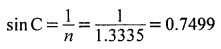∴ C = 48°34′

Question 23.
Refractive index of glass is 1.5 and of diamond is 2.42. Calculate the polarising angle for glass-diamond interface.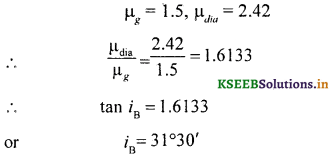Question 24.
A ray of light is incident on the surface of a glass plate of R.I 1.55 at the polarising angle of incidence. Calculate the angle of refraction.
µ = 1.55 tan iB = 1.5500
iB = 57° 10′sin r = 0.5421
∴ r = 32°50

Question 25.
If three fringes are missing in front of the double slit then express ‘λ’ in terms of ‘d’ and ‘D’Question 26.
Calculate the semiangular width of the fifth bright fringe from the central fringe.
For a constructive interference, nλ = d sin θ for fifth bright fringe, n = 5.
sin θ = $$\frac{5 \lambda}{d}$$
∴ θ = sin-1 = $$\left(\frac{5 \lambda}{d}\right)$$

Question 27.
If the distance between the double slit and the screen is doubled and width of the double slit is halved then what will be the new fringe width.Hence New fringe width will be four times the old fringe width.
Percentage increase in the fringe width width = $$\frac{\beta^{\prime}-\beta}{\beta} \times 100$$ = 300%

Question 28.
A bright fringe is formed in front of one of the double slits. What will be the fringe width of the interference pattern?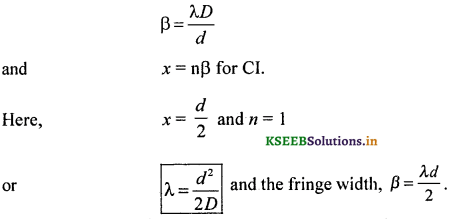Question 29.
A light of intensity 100 Wm2 strikes a film at 57°. Find the intensity of transmitted light.
I = I0 cos2 θ; cos2 θ(0.5446)2 = 0.2966
∴ I = 100 × 0.2966 = 29.66 Wm-2

Question 30.
Two polaroid sheets are placed in such way that one is inclined at an angle of 47° and the second at 63°. Tf the intensity of incident light is 1000 Wm-2, then find the intensity of light at the transmitted ends of the sheets.
I = Io cos2 0
I = 1000 × cos2 47°
= 1000 × (0.6807) f
= 680.7 Wm-2 through crystal (1)
For the second crystal,
I’ = I cos2 63°
= 680.7 × (0.4560)2
= 140.3 Wm-2Question 31.
A ray of light is incident on the surface of a glass plate of R.I 1.55, at the polarising angle of incidence. Calculate the angle of refraction.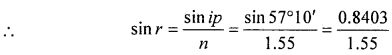The size reduces to half according to the relation size ≈ $$\frac{\lambda}{d}$$; where d = 2d ‘ and size = half the original size. The angular size of central maximum
θ = $$\frac{n \lambda}{d}$$ and intensity of central maximum is proportional to cos2θ.